# Mathematics of Neural Networks

### Mathematics Underpins the World Around Us!Published
May 31, 2017
by
Rickesh Bedia
Categories

This blog article contains a few worked examples and some exercises for you to try yourself. For maximum benefit, find a piece of paper and a pen and work through the problems as you go.

To recap on the fundamentals of Neural Networks, click here, in my Deep Learning Blog. I also covered the basis of the maths behind the neural network. In this blog, I'm going to go into more detail with the Maths, and attempt to explain some higher level concepts.

We could use a pre-build library or framework (examples), but no, we want a true understanding of what is happening. We are going to model our own neural network.

## Our Very Own Neural Network

The neural network we are going to model is a very simple case. It has 2 inputs (i1, i2) 1 hidden layer with 2 neurons (h1, h2) and 2 outputs (o1, o2).

This neural network could be modelling how to get from [1, 2] to [3, 4]. Or, say you gave a word a numerical value based on the position of the letter in the alphabet (a = 1, b = 2, ..., z = 26). You may want to predict the next words for a keyboard. For example, what are the next two words after "How are"? "How" has value 8 + 15 + 23 = 46. "are" has value 1 + 18 + 5 = 24. The inputs are 46 and 24, and you want to train your neural network to output "you today", with values 61 and 65. You then have a problem of decoding, as 65 could represent "today" or "wori", which although is not a word, still has the correct value.

In order to create a neural network you need the following: (The values in the brackets relate to the above 2 -2 -2 neural network.)

1. A set of inputs (1, ..., n) (i1 i2)
2. A set of outputs (1, ..., m) (o1 o2)
3. Number of Hidden Layers (1)
4. Number of Neurons in each Hidden Layer (2 - h1 h2)

Note: n does not need to equal m. Each Hidden Layer doesn't need to have the same number of neurons.

Optional properties:

1. Bias for each neuron in the hidden layer(s) (b1 b2) and output layer (b3 b4)
2. Weights of the Bias's in the hidden layer(s) (v11 v12) and output layer (v21 v22)
3. Weights connecting neurons (w1 w2 w3 w4 - for input to hidden, w5 w6 w7 w8 - for hidden to output)

#### Why are these properties optional?

From the calculations you will see that the bias is only needed to calculate the net of the neuron. Once we start differentiating, the bias doesn't depend on anything, so it is always evaluated to 0. A similar case is said for the weights of the bias.

In a neural network, as it is trained, the weights are updated so minimise the error between the inputs and your outputs. Therefore the weights are constantly being updated. Therefore, by specifying the starting weights, you are providing a starting point as you have no idea what the final value of the weight may be. There is a high possibility that the value is negative. You may as well have a random number between 0 and 1.

## Why do I want to go near Maths?

You're probably wondering to yourself, what possible reason could I have for going near Maths again? Didn't I leave that life behind at GCSE/A-Level where by the end of the course, their were more letters than numbers? An English Degree probably had less letters than that. However, Maths is very important, and I am not biased because I am a Maths Graduate. For programming in general, Maths allows you find optimizations for your processes, and gives you the obvious benefit when doing calculations. You don't need Maths to be a great programmer but it certainly helps.Now, for Machine Learning, I would argue that Maths is more important. Looking at Google's server room, there are hundreds of servers running the calculations needed for search and all their other processes. Remember, Google employs very smart employees to make sure that their programs are running as efficiently as possible given their resources. Imagine, how many servers Google would need without a Maths basis.

You and I are not Google or Facebook or Microsoft. We need Maths for the Algorithms, building the Neural Networks, Linear Algebra and different Algorithms based on the data we are trying to model.

## Our First Neural Network

Lets take this one step at a time. First we are going to focus on the hidden layer. Lets calculate the value of h1.

As we can see, h1 depends on i1, with weight w1, i2, with weight w3, and b1, with weight v11. To calculate the net value of h1, we multiply the value of the neuron and its weight. This can be formulated by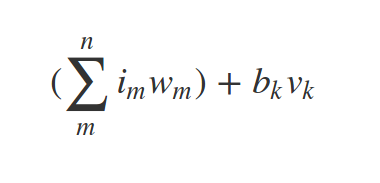Therefore,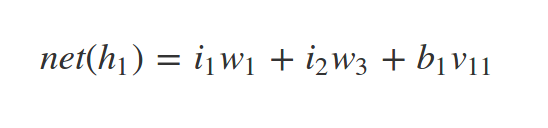We then put this value of h1 into the Sigmoid Function. From my Deep Learning article, a sigmoid neuron outputs a smooth continuous range of values between 0 and 1. As exponential functions are similar to handle mathematically and, since learning algorithms involve lots of differentiation (Spoiler Alert!), choosing a function that is computationally cheaper to handle is great.

The sigmoid function is defined to be: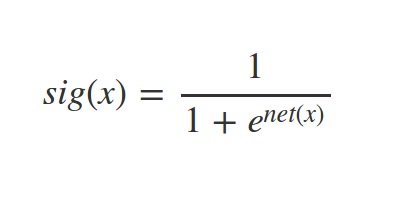Therefore the output of h1 is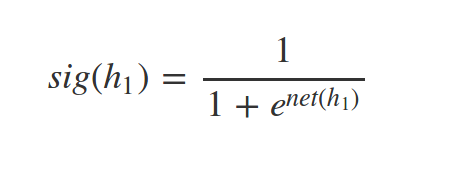We can repeat this same process with h2. h2 depends on i1, with weight w2, i2, with weight w4, and b2, with weight v12.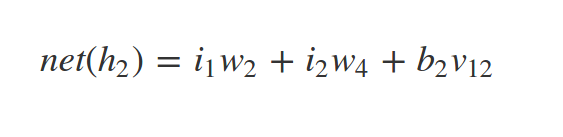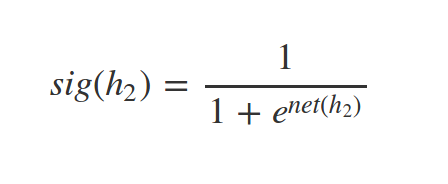If you feel you have understood up to this point, firstly congratulations, and secondly see if you can work out the net() and sig() for o1 and o2.

WARNING: DO NOT READ PAST THIS POINT IF YOU WANT TO ATTEMPT THE ABOVE EXERCISE!!

HINT!:

The results for o1 and o2 are as follows:

THIS IS YOUR LAST CHANCE TO TRY YOURSELF BEFORE I REVEAL MY SOLUTIONS: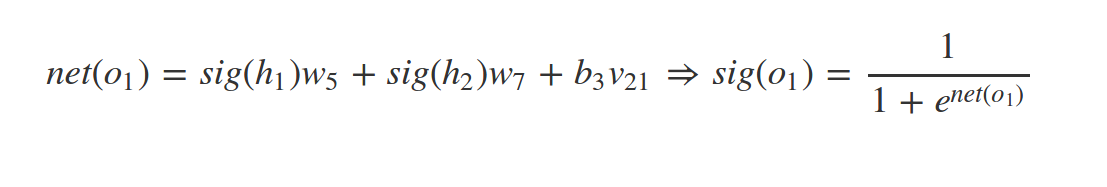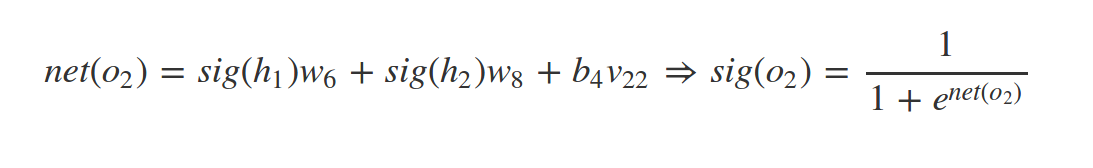Note that we use the sigmoid value of h1, (sig(h1)), not the Net value.

Now that you have the solutions, I'm sure you can see that working out the sigmoid isn't nearly as scary as you imagined it might be. Just a simple case of plugging values into formulae.

So we have our two sigmoid values for the outputs, o1 and o2. We can then compare sig(o1) and sig(o2) to the outputs we chose, say target(o1) and target(o2). To work out the total error, we use the Euclidean norm.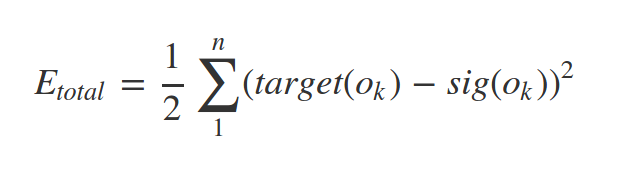Therefore our Total Error is: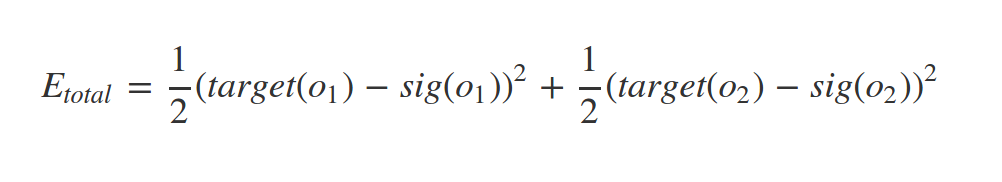## Maths is Fun, I Promise!

Time for the fun part, partial differentiation. I guess it depends on your definition of fun but let's just assume that we have the same definition. For the next section, all you need is some basic knowledge of partial differentiation and maybe a little chain rule. For those of you with a Maths background or know some partial differentiation, you may be able to figure out why, from this point the bias becomes irrelevant.

In the next section I am going to throw a lot of Maths your way. If you understand the derivations, awesome, if not, that's also perfectly fine. You can simply use the results and your neural network model will be no less special.

We defined the our total error to beI want to see how the sig(o1) affects the total error. This is a simple way to think of partial differentiation. As you can see from Etotal, it depends on 4 arguments, target(o1), target(o2), sig(o1) and sig(o2). Finding the Partial Derivative means differentiation on only one variable, not all the variables. (This isn't a mathematically sound definition but I find it helps to think of it in this way.)

Naturally the question is, what is differentiation? Differentiation is the sensitivity of change in the function with respect to it's arguments.

Differentiation is a massive subject in Mathematics, so for this article I am not going to go into how to differentiate. There are many resources online on learning to differentiate. I highly recommend working your way through the Khan Academy course (the first and last links especially), split into easily digestible bitesize chunks.

Let us see how sig(o1) affects the total error. As you can from the equation for Etotal, on the left hand side of the equal, the equation after the '+' doesn't depend on sig(o1) as an argument so this is immediately 0. Therefore we have: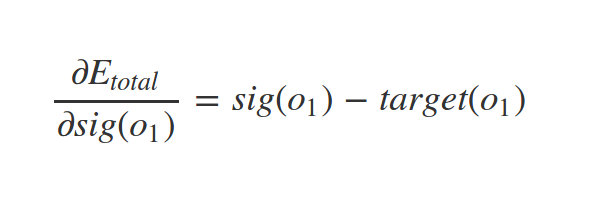Similarly, sig(o2) affects the total error: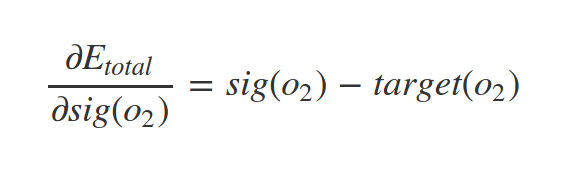We calculated these results using partial differentiation (ignoring part of the equation that does not depend on our argument) and the chain rule to get from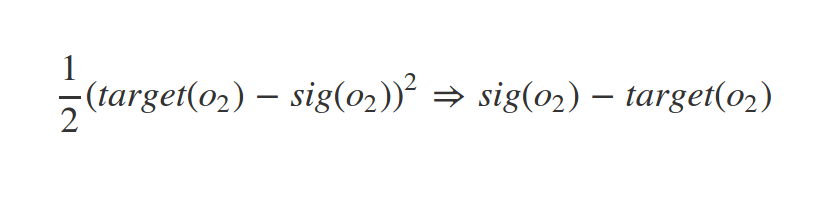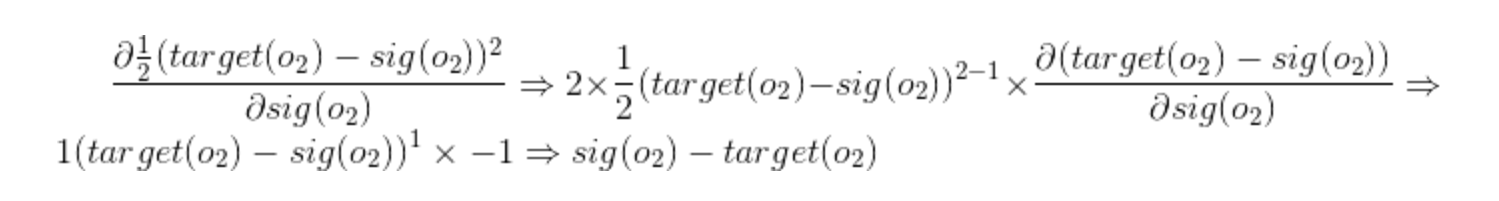(Not the worst but could be prettier)

That's the basics, from here on out, I am simply going to give the results but once you have learned about partial derivatives and the chain rule, I encourage you to figure out these results yourself.

## Updating Weights! Unlike my weight, these may go down as well as up!

The aim of the this section is to see how the weights affect the total error.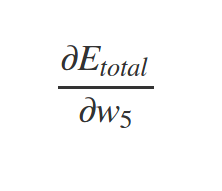You're probably looking at Etotal and thinking, "Etotal doesn't take w5 as an argument so the answer is 0, that was easy!" Before you pat yourself on the back for a great observation, you wouldn't have arrived at the correct conclusion. We have already seen that Etotal depends on sig(o1). sig(o1) depends on net(o1). net(o1) depends on w5. So Etotal does depend on w5.

Therefore to find how Etotal depends on w5 the partial derivative we need to calculate is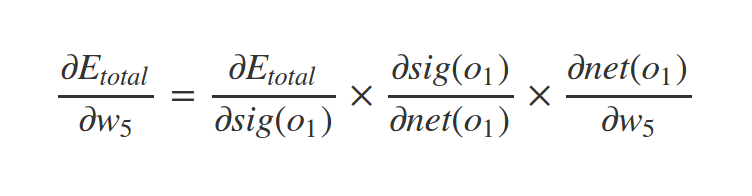If you remember multiplying fractions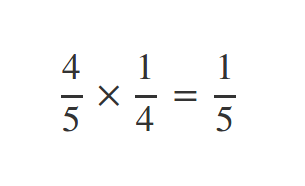you know you can cancel the 4's. You can think of the equation above in a similar way and after "cancelling", you are back to(You are not strictly cancelling so unless you want a lecture on Maths from one of your Mathematically inclined friends, I wouldn't tell anyone that is what you are doing. But just between, you and me, it's cancelling!)

To find how Etotal depends on sig(o1), we calculate the partial derivative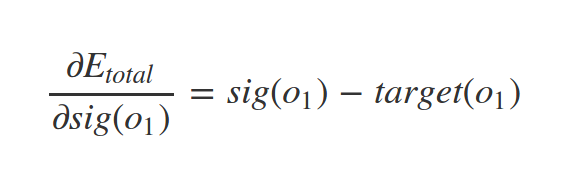To find how sig(o1) depends on net(o1), we calculate the partial derivative of the sigmoid function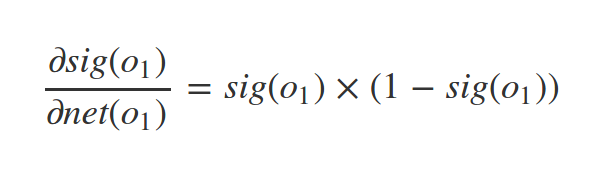This result is particularly tricky. If you have a good understand of differentiation you should try and get this result. If you have no intention of touching this with a 10 foot pole, you can see a solution here, although you may also see your lunch again.

To find how net(o1) depends on w5, we calculate the partial derivative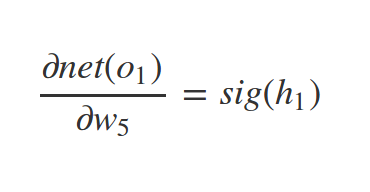Look back at your definition of net(o1) and you'll quickly spot this.

Plugging this into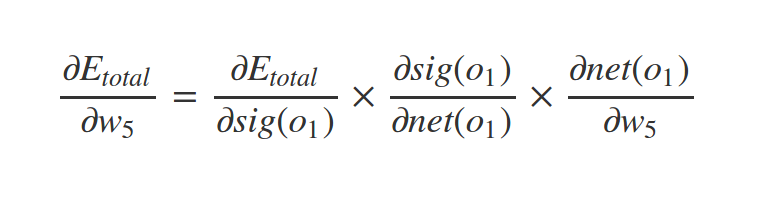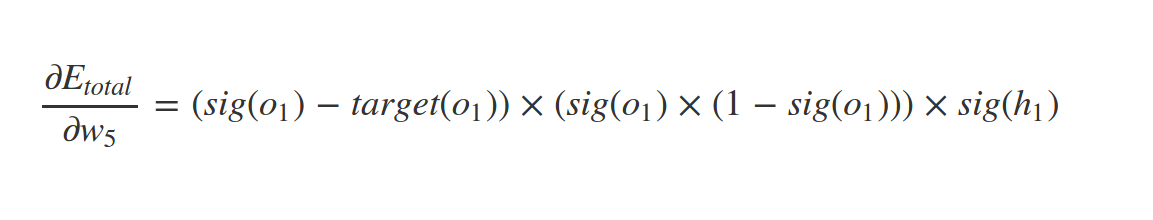Now that we have calculated how w5 affects the total error, lets take a look at the neural network we are modelling, focusing on w5.

You can see that w5 effectively connects the sigmoid value of neuron h1 to neuron o1. Therefore, in the calculation, that is why we see sig(o1) and sig(h1)

The new value of w5, w51, is now (ww50 is the old value)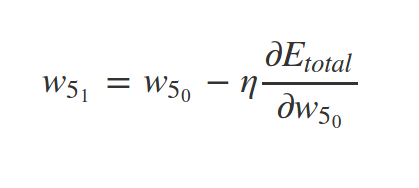Wait a second! What is that weird n and what is it doing in this equation? That "n" is an eta and it's there to represent the learning rate. The higher the learning rate, the quicker your neural network will lower the error to get close to your output. However, the neural network will be less accurate. Normally, the learning rate is set to 1/2.

If you were to take a guess at the equation for w6, what do you think it would be?

You can see that w6 connects the sigmoid value of neuron h1 to neuron o2. Therefore a good guess would be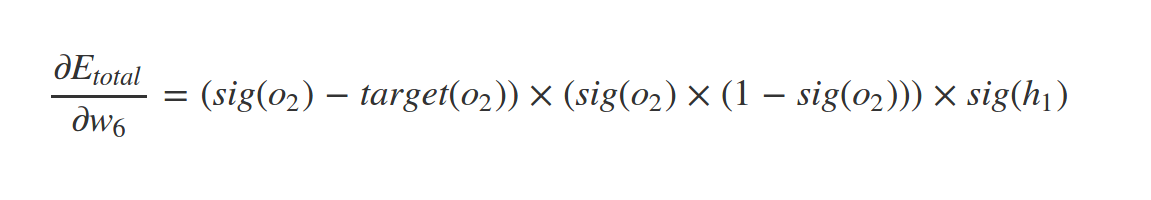See if you can calculate this result, like how we did with w5, noticing how, to find how Etotal depends on w6, Etotal depends on sig(o2) which depends on net(o2) which depends on w6.

Calculate for practice and prove to yourself that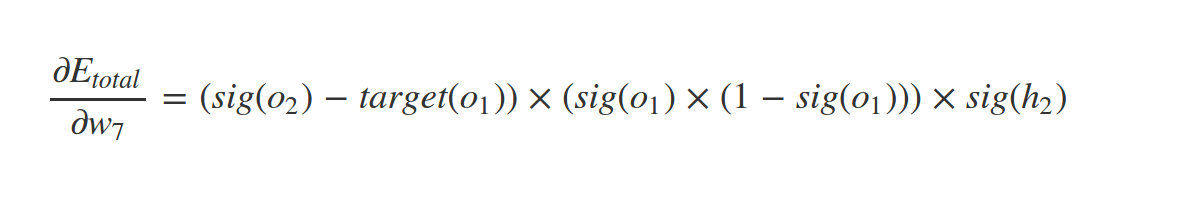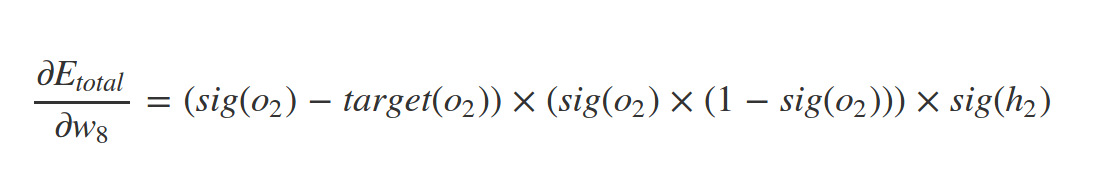Therefore,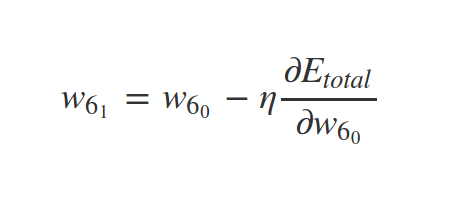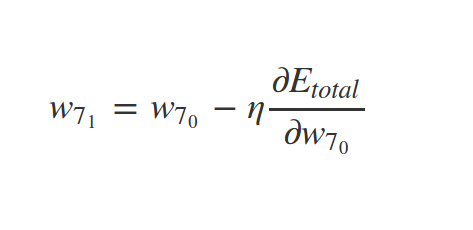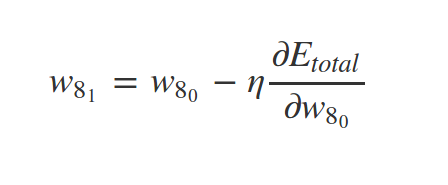The value of eta is the same for every weight in the whole neural network (for weights 1 - 8 not just 5 -8) but I see no reason why they can't be different. It will mean that your neural network weights are learning at different rates, but for some models this may be important. If you care more about one output than the other for example, then in our example, say o2 was more important. The learning rate of w6 and w8 could be higher than w5 and w7.

We have successfully seen how w5, w6, w7 and w8 affect the total error of our network and calculated their new values.

But that is only one layer. How do w1, w2, w3 and w4 affect the total error?

## It's the Final Layer!

Okay, we can see that w1 connects i1 and h1. I'm going to try the same method as we employed for w5. Umm...how does h1 affect the total error?

We know that Etotal depends on sig(o1) and sig(o2). sig(o1) depends on net(o1) and sig(o2) depends on net(o2). net(o1) depends on sig(h1) and net(o2) depends on sig(h1). (Ah, there's the link!) sig(h1) depends on net(h1). net(h1) depends on w1. That was only mildly inconvenient.

Therefore our formula is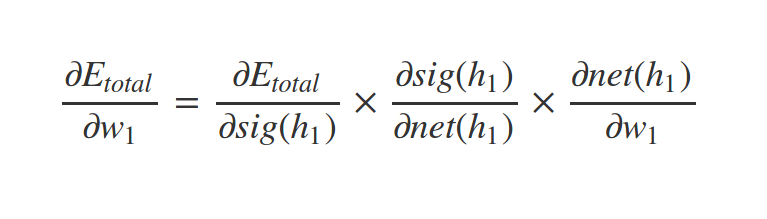where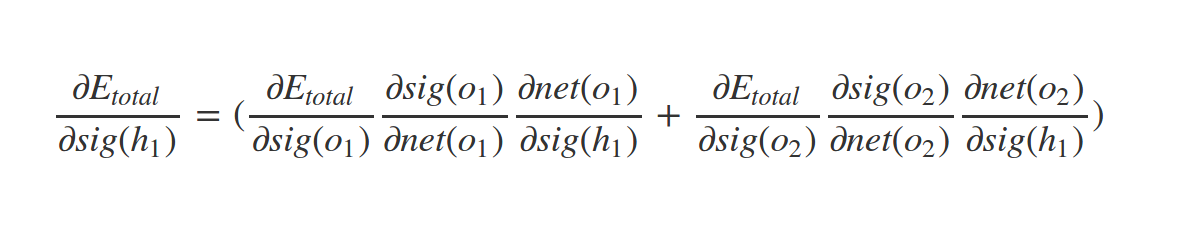To find how Etotal depends on sig(h1), we calculate the partial derivative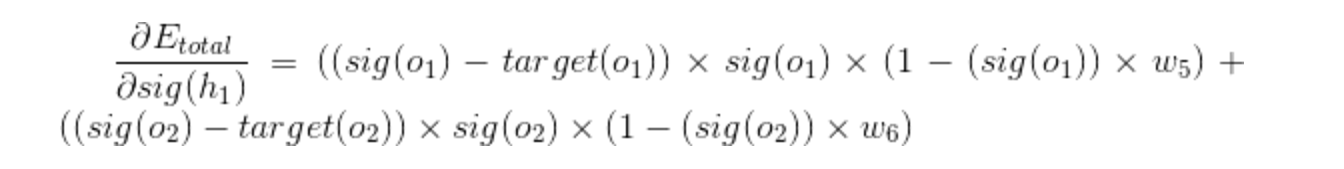To find how sig(h1) depends on net(h1), we calculate the partial derivative of the sigmoid function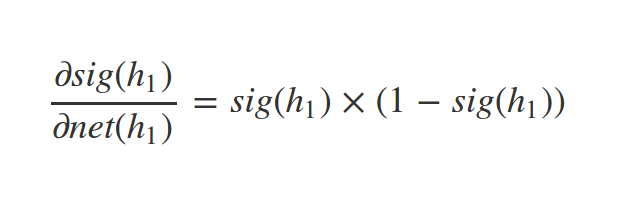To find how net(h1) depends on w1, we calculate the partial derivative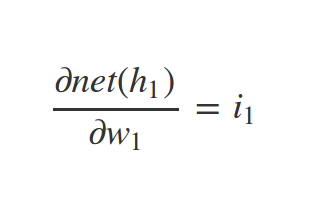Remind yourself of the value of net(h1).

I'll leave the following for you to figure out.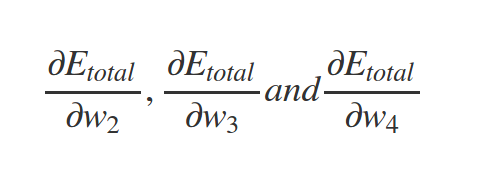Use a similar formulae with the learning rate to find the new values for w1, w2, w3 and w4.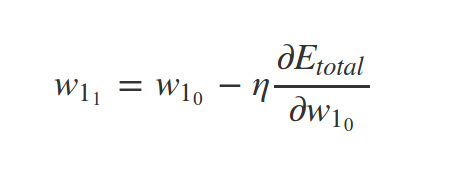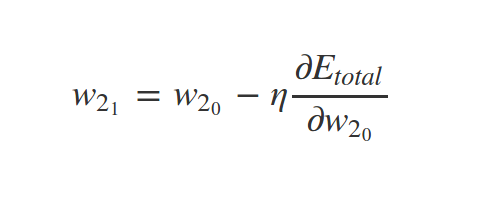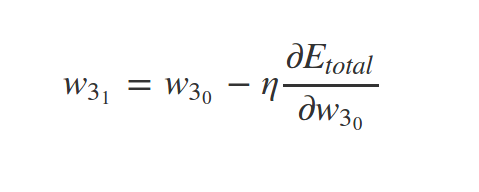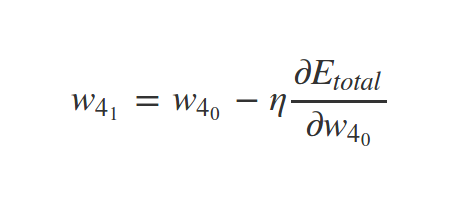So, that's the Maths. If you followed it or not, I'm sure you have a clearer picture of what is happening along those weights. Personally, when going working through the neural network, a visual representation of how the weights are changing, and what affects them helped me.

I'm glad you've made it this far and I hope you now have the Maths ability to write your own neural network in the language of your choice, even Java!

If you found the neural network example puzzling, I would advise you to try working through some neural networks for yourself.

### Remember, Remember the five (plus 1) tips for Neural Networks! Gunpowder, Treason and Plot ....

2. Calculate your nets for each layer and their relevant sigmoid
3. Find the error
4. Differentiate everything or have an educated guess to the updated weights
5. Putting everything together like a jigsaw
6. Relax

Now try, a neural network that is a little difficult. Don't let the bias scare you:

Are you getting the hang of this now? This one might be slightly more challenging (but not for you!):

To become a Maths Master (when it comes to modelling neural networks), your final challenge is:

You'll recognise this next neural network as the one we worked through together. I believe in you, that you can work through this yourself. No Cheating!

How about one more just for fun? Don't tell me you don't find Maths fun now!

Okay so you can calculate a 3 layered neural network. Time to try 4 layers:

As the old saying goes, once you can do 4 layers, you can do an arbitrary number of layers. Doesn't quite roll off the tongue does it.